# Math Equations that changed the World presented with their related GIFs

Mathematics is all around us, and it has shaped our understanding of the world in countless ways. It has contributed in shaping the world as it is now and it has helped the people overcome various normal problems. Presented below are some math equations that categorically the changed the world as we know it.

### 1) Pythagoras’s TheoremImage Courtesy: Tumblr.com

a2 + b2= c2

This theorem is foundational to our understanding of geometry. It describes the relationship between the sides of a right triangle on a flat plane: square the lengths of the short sides, a and b, add those together, and you get the square of the length of the long side, c.

### 2) LogarithmsImage Courtesy: teded.tumblr.com

log xy = log x + log y

Logarithms are the inverses, or opposites, of exponential functions. The logarithm of a number is the exponent to which another fixed value, the base, must be raised to produce that number. Until the development of the digital computer, this was the most common way to quickly multiply together large numbers, greatly speeding up calculations in physics, astronomy, and engineering.

### 3) CalculusImage Courtesy: visualizingmath.tumblr.comImage Courtesy: www.mathdemos.org

∫f(x)dx = g(x)

Naturally, much of science is interested in understanding how things change, and the derivative and the integral sit at the heart of how mathematicians and scientists understand change. The first animation shows the midpoint Riemann sums to approximate the area under the curve. The second animation shows the partial derivative of two functions, z= f(x,y).

### 4) Law of GravityImage Courtesy: blogs.jccc.edu

F = G (m1m2/r2)

Newton's law of gravitation describes the force of gravity between two objects, F, in terms of a universal constant, G, the masses of the two objects, m1 and m2, and the distance between the objects, r. Newton's law is a remarkable piece of scientific history - it explains, almost perfectly, why the planets move in the way they do. Newton stated that his gravity law worked for any two objects with mass - it applies for any motions on the Earth, as well as, any motions in space.

### 5) Euler’s Formula for PolyhedraImage Courtesy: www.mi.sanu.ac.rs

V – E + F = 2

Polyhedra are the three-dimensional versions of polygons. Euler's formula states that, as long as your polyhedron is somewhat well behaved, if you add the vertices and faces together, and subtract the edges, you will always get 2. This will be true whether your polyhedron has 4, 8, 12, 20, or any number of faces.

### 6) Fourier TransformImage Courtesy:1ucasvb.tumblr.com

It is a mathematical transformation employed to transform signals between time domain and frequency domain, which has many applications in physics and engineering.

### 7) Normal DistributionImage Courtesy: www.sumsar.net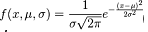The normal curve is used in physics, biology, and the social sciences to model various properties. One of the reasons the normal curve shows up so often is that it describes the behavior of large groups of independent processes.

### 8) Wave EquationImage Courtesy: en.wikipedia.org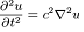This is a differential equation, or an equation that describes how a property is changing through time in terms of that property's derivative. The wave equation describes the behavior of waves - a vibrating guitar string, ripples in a pond after a stone is thrown, or light coming out of an incandescent bulb.

### 9) Maxwell’s EquationsImage Courtesy: en.wikipedia.org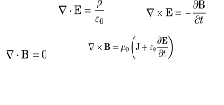This set of four differential equations describes the behavior of and relationship between electricity (E) and magnetism (H). Maxwell’s equations are the foundation of our explanation of how electromagnetism works on a day to day scale.

### 10) Second Law of ThermodynamicsImage Courtesy: htmlsmagicpages.blogspot.com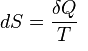The second law of thermodynamics states that the entropy of an isolated system never decreases, because isolated systems always evolve toward thermodynamic equilibrium, a state with maximum entropy. The animation shows an Alpha Type Stirling Engine treated as a second law of thermodynamics.

### 11) RelativityImage Courtesy: www.cleonis.nl

E = mc2

The classic equation E = mc2 states that matter and energy are equivalent to each other. Special relativity brought in ideas like the speed of light being a universal speed limit and the passage of time being different for people moving at different speeds.

### 12) Schrodinger's EquationImage Courtesy: en.wikipedia.org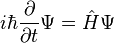This is the main equation in quantum mechanics. As general relativity explains our universe at its largest scales, this equation governs the behavior of atoms and subatomic particles. Quantum mechanics is also necessary for most modern technology — nuclear power, semiconductor-based computers, and lasers are all built around quantum phenomena. The animation shows a A harmonic oscillator in classical mechanics (A–B) and quantum mechanics (C–H). In (A–B), a ball, attached to a spring, oscillates back and forth. (C–H) are six solutions to the Schrödinger Equation for this situation.

### 13) Chaos TheoryImage Courtesy: universe-review.ca

xt+1 = kxt(1-xt)

This equation is a logistic map. It describes a process evolving through time - xt+1, the level of some quantity x in the next time period - is given by the formula on the right, and it depends on xt, the level of x right now. k is a chosen constant. For certain values of k, the map shows chaotic behavior: if we start at some particular initial value of x, the process will evolve one way, but if we start at another initial value, even one very very close to the first value, the process will evolve a completely different way.

### You may also like:

18662   07/07/2014